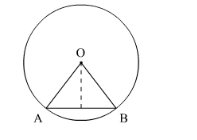# The perimeter of a certain sector of a circle of radiusQuestion:

The perimeter of a certain sector of a circle of radius 5.6 m is 27.2 m. Find the area of the sector.

Solution:

We know that the area A of a sector of circle of radius r and arc length l is given by

$A=\frac{1}{2} l r$

Let OAB is the given sector. Then,

Perimeter of sector OAB = 27.2 m

OA + OB + arc AB = 27.2 m

5.6 + 5.6 + arc AB = 27.2 m

11.2 + arc AB = 27.2 m

$\operatorname{arc} \mathrm{AB}=16 \mathrm{~m}$

So, $l=16 \mathrm{~m}$Now substituting the value of r and l in above formula,

$A=\frac{1}{2} \times 16 \times 5.6$

$=44.8 \mathrm{~m}^{2}$